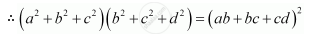Share

# If A, B, C and D Are in Geometric Progression. Show that (A2 + B2 + C2) (B2 + C2 + D2) = (Ab + Bc + Cd)2 . - Mathematics

#### Question

If a, b, c and d are in G.P. show that (a2 + b2 + c2) (b2 + c2 + d2) = (ab + bc + cd)2 .

#### Solution

abcd are in G.P.

Therefore,

b2 = ac … (2)

c2 = bd … (3)

It has to be proved that,

(a2 + b2 + c2) (b2 + c2 + d2) = (ab + bc – cd)2

R.H.S.

= (ab + bc + cd)2

= (ab + ad cd)2 [Using (1)]

= [ab + d (a + c)]2

a2b2 + 2abd (a + c) + d2 (a + c)2

a2b2 +2a2bd + 2acbd + d2(a2 + 2ac + c2)

a2b2 + 2a2c2 + 2b2c2 + d2a2 + 2d2b2 + d2c2 [Using (1) and (2)]

a2b2 + a2c2 + a2c2 + b2cb2c2 + d2a2 + d2b2 + d2b2 + d2c2

a2b2 + a2c2 + a2db× b2 + b2c2 + b2d2 + c2b2 + c× c2 + c2d2

[Using (2) and (3) and rearranging terms]

a2(b2 + c2 + d2) + b2 (b2 + c2 + d2) + c2 (b2c2 + d2)

= (a2 + b2 + c2) (b2 + c2 + d2)

= L.H.S.

∴ L.H.S. = R.H.S.Is there an error in this question or solution?